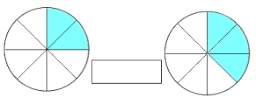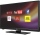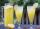# Fractions 4

How many 2/3s are in 6?

n =  9

### Step-by-step explanation:

$n=6\mathrm{/}\frac{2}{3}=9$Did you find an error or inaccuracy? Feel free to write us. Thank you!Tips to related online calculators
Need help to calculate sum, simplify or multiply fractions? Try our fraction calculator.

## Related math problems and questions:

• How many 3How many hours the Andersons watched TV in all Wednesday 3/1 hr Thursdays 2/3 hr Friday 4/5 hr Saturday 3/4 hrHow many 1/4 cup servings are in 2 and 1/3 cups of lemonade?
• Mixed divided with fracThere are 2 2/3 pizzas. How many people are sharing when each has 2/3 of pizza?
• Evaluate mixed expressionsWhich of the following is equal to 4 and 2 over 3 divided by 3 and 1 over 2? A. 4 and 2 over 3 times 3 and 2 over 1 B. 14 over 3 times 2 over 7 C. 14 over 3 times 7 over 2 D. 42 over 3 times 2 over 31
• Fractions and mixed numerals(a) Convert the following mixed numbers to improper fractions. i. 3 5/8 ii. 7 7/6 (b) Convert the following improper fraction to a mixed number. i. 13/4 ii. 78/5 (c) Simplify these fractions to their lowest terms. i. 36/42 ii. 27/45 2. evaluate the follow
• The book 4Mr. Kinion read 3 3/4 chapters in his book on Monday. He then read 2 4/6 more chapters on Tuesday. How many chapters has he read so far?
• A quotientWhat is the quotient of 3/10 divided by 2/4 as a fraction?
• Numbers divisionWith what number should be divided mixed number 2 3/4 to get 11/12?
• RunningJoanne ran 8 1/2 Kyleigh 1 2/3 and John 6 3/4 miles in one day. How many miles did the girls run in 3 days?
• Difference mixed fractionsWhat is the difference between 4 2/3 and 3 1/6?
• Hours 3How many hours are in 3 2/3 days?
• 5 2/55 2/5 hours a week  mathematics,  3 3/4 hours a week   Natural sciences, 4 3/8 hours a week  Technology . how many hours does  he spend on social sciences if he spend 17 1/2 hours a week for the four subject?
• EvaluateThe division of numbers $a and$b increase by-product of the numbers $c and$dAdd two mixed fractions: 2 4/6 + 1 3/63 3/4 + 2 3/5 + 5 1/2 Show your solution.How many fifths are there in four wholes? How many 1/5 are there in 4?Find the quotient of 3/4 and 1/4.